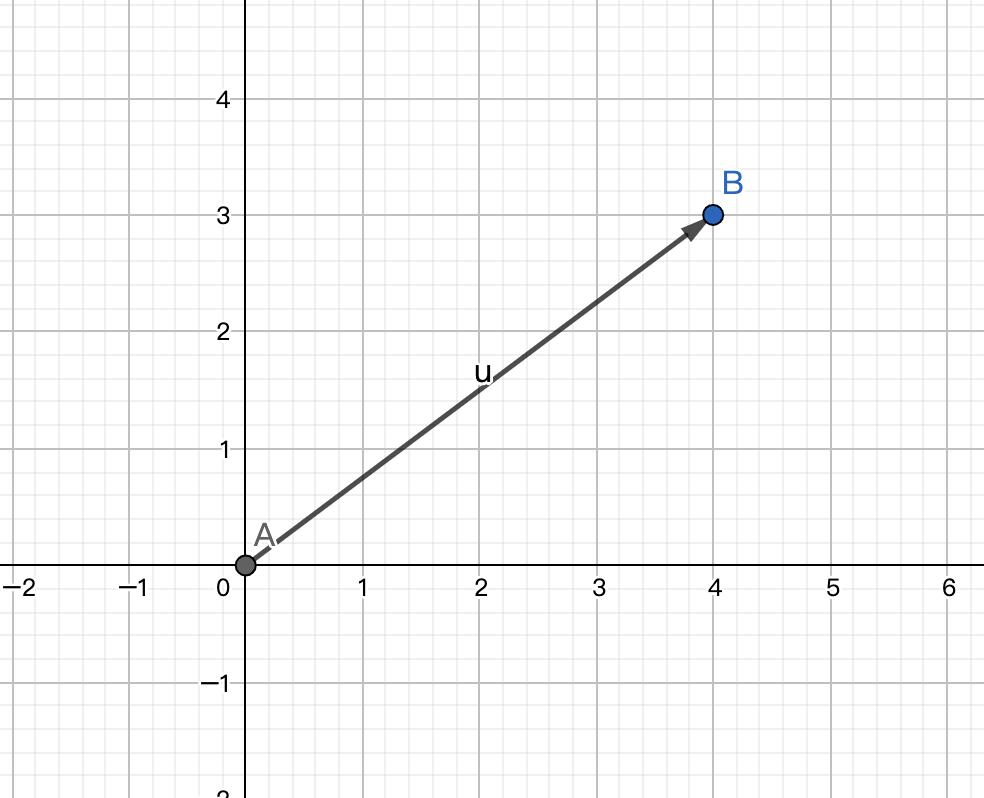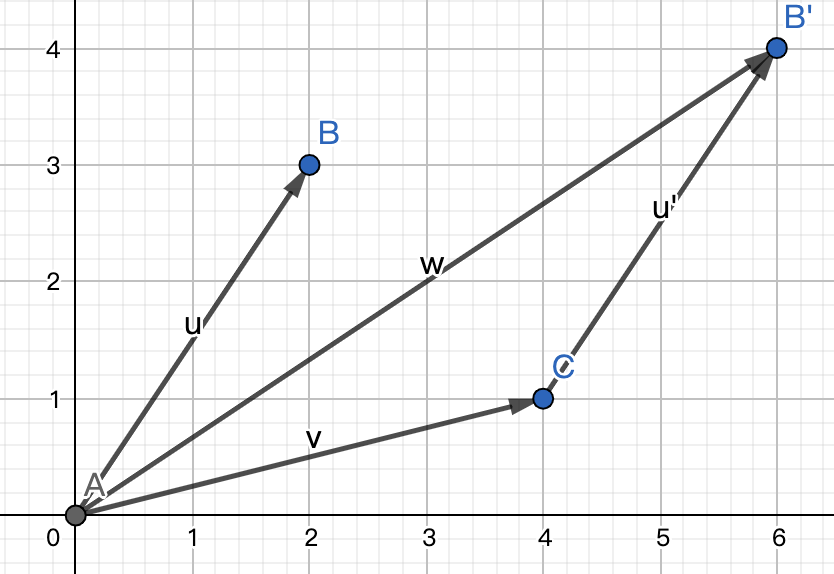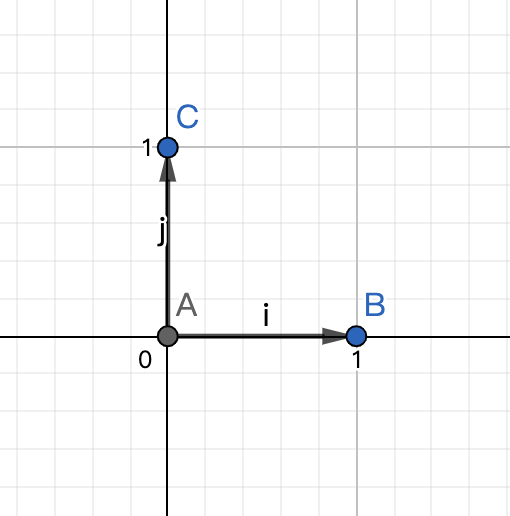# 线性代数(Linear Algebra)学习笔记

## 向量(Vectors)## 向量的基础运算## 基向量## 线性变换

• 直线在变化后仍保持为直线，不能有所弯曲
• 原点必须保持固定

## 矩阵

### 矩阵的定义

$\hat{i}$变化后变为了$\begin{bmatrix} 1\ 3 \end{bmatrix}$ $\hat{j}$变化后变为了$\begin{bmatrix} -2\ 0 \end{bmatrix}$ 则向量$\begin{bmatrix} x\ y \end{bmatrix}$的变换过程为：